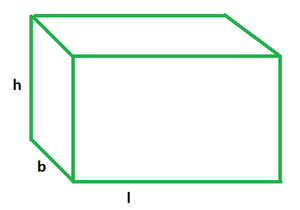# Surface Area of a Rectangular Prism Formula

• Last Updated : 22 Mar, 2022

Such a polygon that has two parallel bases is called a prism. So a rectangular prism is one whose two parallel bases are rectangular. Apart from those two, it has four rectangular faces as well. A rectangular prism can be regarded as a cuboid. It is shown as follows:### Surface Area of a Rectangular Prism

The surface area of a rectangular prism is equal to the sum total of the surfaces areas of all its faces. Now since it is composed of all rectangular faces, and the area of a rectangle is given by the product of its length and width, add up all the products of lengths and breadths to conjure up the surface area of a rectangular prism.

Formula

A = 2(lb + lh + bh)

where,

l, b and h denote the length, breadth and height of rectangular prism

### Sample Problems

Question 1. Find the surface area of a rectangular prism if its length, breadth and height are 4 m, 5 m and 8 m.

Solution:

Given: l = 4 m, b = 5 m and h = 8 m

SA = 2(lb + lh + bh)

= 2(4×5 +4×8 + 5×8)

= 2(20 + 32 + 40)

= 2(92)

A = 184 m2

Question 2. Find the surface area of a rectangular prism if its length, breadth and height are 2 m, 7 m and 10 m.

Solution:

Given: l = 2 m, b = 7 m and h = 10 m

SA = 2(lb + lh + bh)

= 2(2×7 + 2×10 + 7×10)

= 2(14 + 20 + 70)

= 2(104)

A = 208 m2

Question 3. Find the surface area of a rectangular prism if its length, breadth and height are 9 m, 6 m and 7 m.

Solution:

Given: l = 9 m, b = 6 m and h = 7 m

SA = 2(lb + lh + bh)

= 2(9×6 + 7×9 + 6×7)

= 2(54 + 63 + 42)

= 2(159)

A = 318 m2

Question 4. Find the surface area of a rectangular prism if its length, breadth and height are 7 m, 6 m and 5 m.

Solution:

Given: l = 7 m, b = 6 m and h = 5 m

SA = 2(lb + lh + bh)

= 2(7×6 + 7×5 + 6×5)

= 2(42 + 35 + 30)

= 2(107)

A = 214 m2

Question 5. Find the surface area of a rectangular prism if its length, breadth and height are 8 m, 5 m and 9 m.

Solution:

Given: l = 8 m, b = 5 m and h = 9 m

SA = 2(lb + lh + bh)

= 2(8×5 + 8×9 + 5×9)

= 2(40 + 72 + 45)

= 2(157)

A = 314 m2

My Personal Notes arrow_drop_up